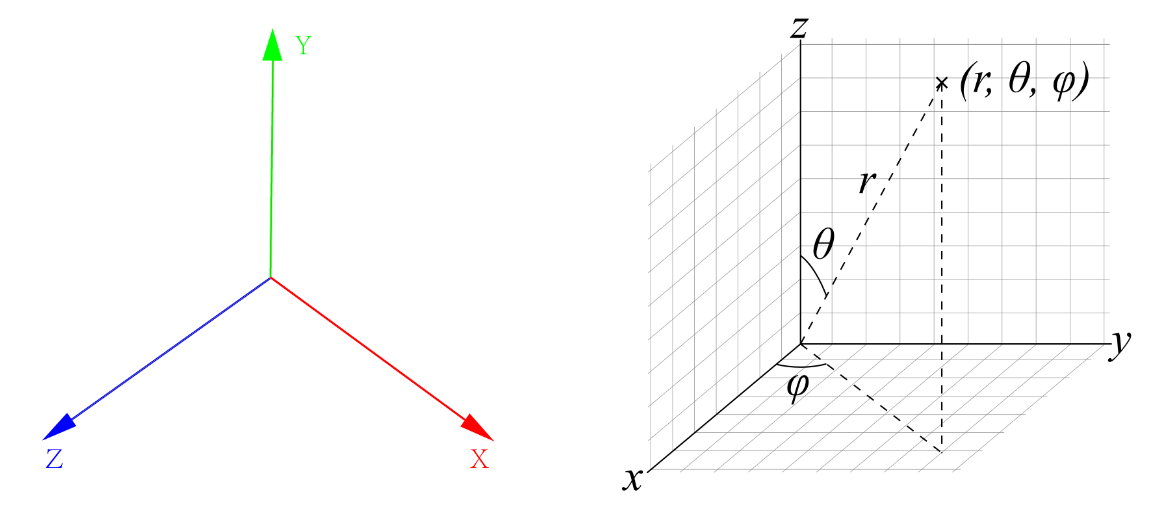# VTK 示例：坐标转换x = r * std::sin(theta) * std::sin(phi)
y = r * std::cos(theta)
z = r * std::sin(theta) * std::cos(phi)


theta = glm::radians(theta);